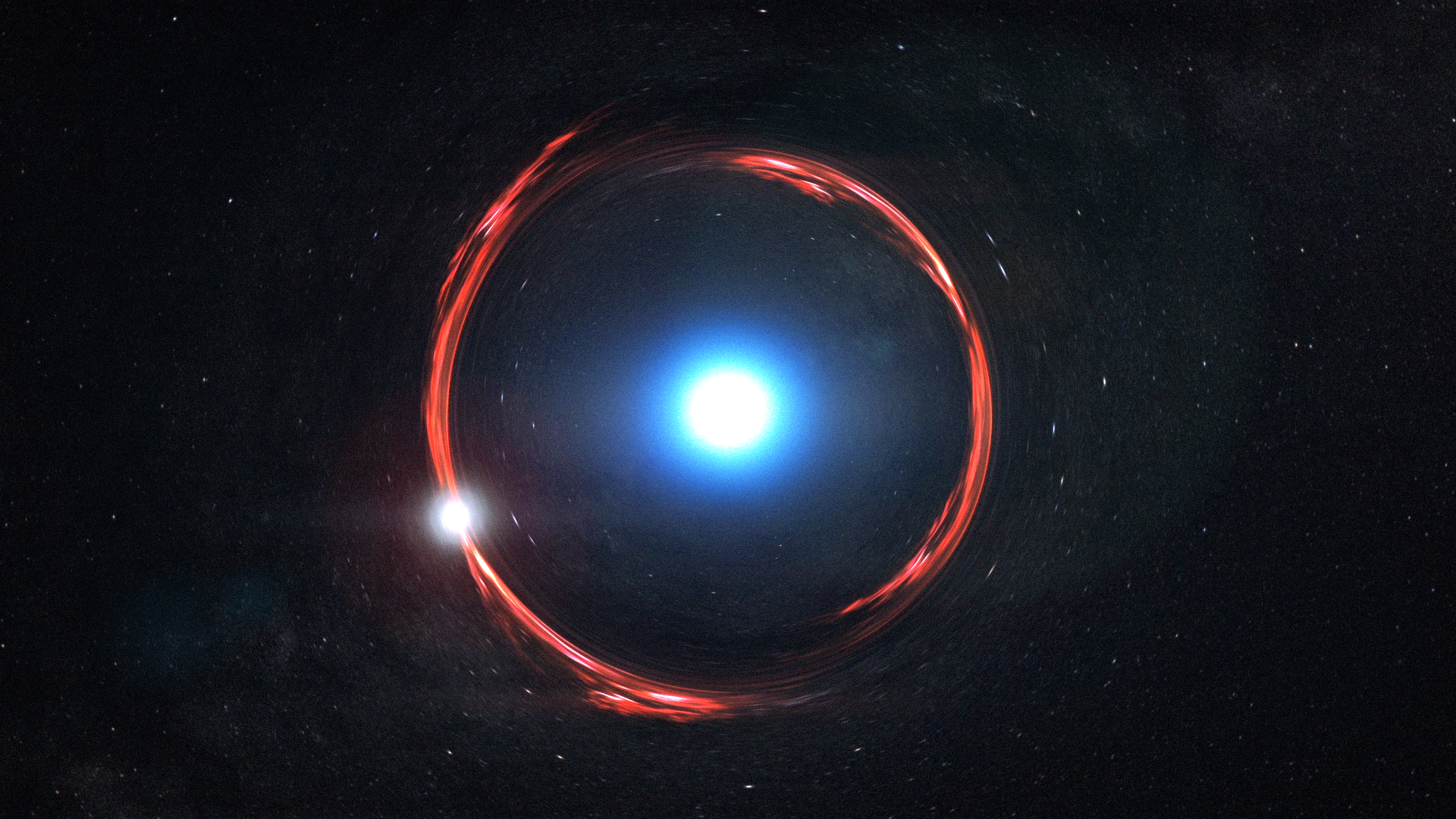## GRAVITATIONAL LENSING

WHAT IS GRAVITATIONAL LENSING?

A gravitational lens is a distribution of matter (such as a cluster of galaxies) between a distant light source and an observer, which is capable of bending the light from the source as the light travels towards the observer.

Gravitational lensing is one of the experimental evidence that objects having mass bends the spacetime. Einstein predicted that if his general theory of relativity is right then light should take a curve path while passing close to any star or galaxy.

The general theory of relativity is the modified version of Newton’s gravitational law. With the help of general relativity, Einstein showed that objects having mass like you, me, earth, sun, galaxies, etc. change the geometry of spacetime.

WHAT THE BENDING OF SPACETIME ‘LOOKS’ LIKE?

To understand this clearly, let’s consider an analogy. Imagine a thin rubber sheet and stretch it such that it shouldn’t be curved. Place a ball on this rubber sheet, you can observe that the ball has curved or ‘bend’ the sheet due to its weight.

Now you can compare the ball with the sun and rubber sheet with spacetime. Hope you get the point. Do note that in this demonstration the rubber sheet bends due to the ball’s weight. But this shouldn’t consider the reason in case of real sun bending spacetime. Because there is no such downward force acting in space, instead it’s the mass which causes the bending of spacetime.

Therefore, the analogy has many disadvantages, so they shouldn’t be taken too seriously. It’s just to understand or imagine what bending of spacetime means.

CAN THE SPACETIME BEND LIGHT?

We know that light always travels in a straight line in space. Then how can it be bend as per Einstein? Well… the answer lies in the change in geometry of spacetime. To let you physically imagine this, let me give you the same example with a different angle.

Stretch your rubber sheet and now throw a small marble across it, it will go straight. Now place a heavy ball and again try to throw the marble at a specific angle. This time you will observe that due to the bending of your rubber sheet i.e. spacetime, the marble turns and change its regular path.

Now imagine a massless photon in place of the small marble. You may also perform another experiment. Draw horizontal lines on the rubber sheet at equal intervals. These lines will be denoting the rays of light coming from infinity.

Now when you place your heavy ball, you will observe that the lines appeared to be bending near the curvature. The angle at which the lines (or light in this case) appear to be bending depends upon the mass of the object.

IS THE THEORY OF GENERAL RELATIVITY RIGHT?

To prove Einstein’s theory of general relativity correct, an observation was made at a distant light source. Let’s imagine you are doing this observation to get that scientist feeling for the time being.

You are watching a distant star via a telescope and there is no matter between your telescope and the star. You know that light travels in a straight line. So light from the star enters your telescope and you see one image of that star.

Now imagine there is a galaxy in between your telescope and the distant star. Since the galaxy has huge mass, it should have to bend the fabric of spacetime and light must bend at a specific angle.

Thus, you will observe more than one image of the same star at different locations. And guess what? That’s what scientists observed. Definitely, no star can be at two positions simultaneously, it’s just an illusion due to the bending of spacetime.

Since the galaxy is behaving like a lens, such a phenomenon is called gravitational lensing. Now you can definitely mathematically calculate the mass of the galaxy, angle by which the light appears to bend, etc. and can also compare with your telescopes to see whether the theory is right or not.

Guess what? The mathematical prediction matches with the experiments, but here’s one twist which made the scientific community baffled. The calculated mass of the galaxy did not match with observation. There was some extra mass which they were getting through the experimental results.  So who is contributing this extra mass? Are the scientists unable to see something? that’s what I will be answering in my next blog post. However, I would like you to guess the answer by commenting below and subscribe my blog to know the answer in my next blog post 🙂

1.1.